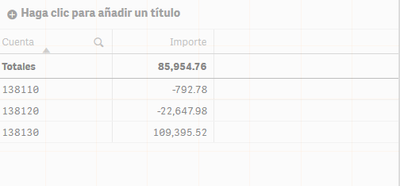# New to Qlik Sense

Discussion board where members can get started with Qlik Sense.

Announcements
BI & Data Trends 2021. Discover the top 10 trends emerging in today. Join us on Dec. 8th REGISTER
cancel
Showing results for
Did you mean:
HighlightedContributor III

## Get Positive Value in KPI

Hi, I am facing a problem to fetch only the Positive Value from the Difference

The Pivot table is this

 Model Number Forecast Billing Quantity Sum(Forecast)-Sum(Billing Qunatity) Model-A 10 8 2 Model-B 60 116 -56 Model-C 775 456 319 Model-D 40 124 -84 Model-E 52 59 -7 Model-F 240 650 -410

I want to Show the value "Sum(Forecast)-Sum(Billing Qunatity)" in a KPI but only the sum of positive value but unable to do do the KPI gives : 2+(-56)+319+(-84)+(-7) +(-410) =  -236. The Output I want is should be ( 2+319 = 321) i.e only the positive value.

Can anyone help me with that how can i achieve this Output in KPI

Thanks

Rishabh

Labels (3)

• ### Qlik Sense

1 Solution

Accepted Solutions
HighlightedPartner

You can use SET analysis to accomplish this. I've used your data in an QVF for presentation below.Try this expression:

``````=Sum({<[Model Number]= {"=sum(Forecast)>=sum([Billing Quantity])"} >}Forecast)
-
Sum({<[Model Number]= {"=sum(Forecast)>=sum([Billing Quantity])"} >}[Billing Quantity])``````

See attached qvf

Plees ekskuse my Swenglish and or Norweglish spelling misstakes
7 Replies
HighlightedMVP
You can put a check before final summation, something like:
Sum(If(yourvalue>0, yourvalue))
HighlightedPartner

You can use SET analysis to accomplish this. I've used your data in an QVF for presentation below.Try this expression:

``````=Sum({<[Model Number]= {"=sum(Forecast)>=sum([Billing Quantity])"} >}Forecast)
-
Sum({<[Model Number]= {"=sum(Forecast)>=sum([Billing Quantity])"} >}[Billing Quantity])``````

See attached qvf

Plees ekskuse my Swenglish and or Norweglish spelling misstakes
HighlightedCreator

sum(aggr(if(sum(Forecast)>sum(BillingQuantity),Sum(Forecast)-Sum(BillingQuantity)),Forecast,BillingQuantity))

HighlightedCreator II

Thanks and Regards, Swarnendu Haldar.

HighlightedContributor III

Yeah It works...

Thanks you very much @Vegar🙂

HighlightedContributor III

Is this also Possible to get positive value only of this expression

 Model Number Forecast Billing Quantity Forecast_2 Left Quantity (Sum(Forecast) - sum(Billing Quantity)) - (sum(Forecast_2)+sum(Left_Quantity)) Model-A 10 8 48 0 -46 Model-B 60 116 205 0 -261 Model-C 775 456 1832 0 -1513 Model-D 25 2 2 8 13 Model-E 52 59 2303 0 -2303 Model-F 80 10 20 3 47

Can we also get positive value of  following expression :  i.e. 13+47 = 60

(Sum(Forecast) - sum(Billing Quantity)) - (sum(Forecast_2)+sum(Left_Quantity))

HighlightedContributor III